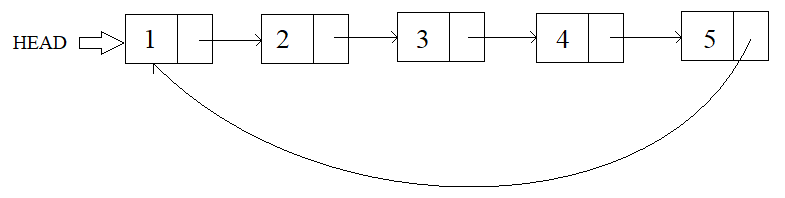# Deletion In Circular Linked List

Posted: 16 Jul, 2020
Difficulty: Easy

## PROBLEM STATEMENT

#### You have to write a function that finds the given key in the list and deletes it. If no such key is present, then the list remains unchanged.

##### For Example :
``````This is a visualization of the Circular Linked List, represented by:
1 2 3 4 5 -1
``````##### Note :
``````The Circular Linked List before/after deletion may happen to be empty. In that case, only print -1.

All integers in the list are unique.
``````

##### Input format :
``````The first input line contains the integers present in the circular linked list in order.

The second input line contains a single integer 'key', the key to be deleted.
``````
##### Remember/Consider :
``````-1 marks the end of the linked list however, the tail of the linked list would be pointing to the head making it circular in nature.
``````
##### Output format :
``````The only output line contains the updated circular linked list post deletion.
``````
##### Constraints :
``````0 <= N <= 10 ^ 5
1 <= key <= 10 ^ 5

Where 'N' is the length of the Circular Linked List.

Time limit: 1 sec
``````Approach 1

The idea is to use a recursive approach to find the given key and then remove that node. The recursive idea is very clear that we will traverse through the circular linked list, and we will check if the value of the node is equal to the given key.

We will define a function deleteNodeHelper(root, key, head) to remove the key in which the head is the starting node of the linked list, and we will send root as the next node of the head node.

Working of the Recursive function:

1. If the root is equal to head, then we have traversed the whole linked list and have not found the given key. So, we will return head. This will act as the base case of the recursive function.
2. We will check if the value of the node is equal to the key. We have found the node with the given key, and we have to remove this node. So, we will return the next node of the root.
3. Now, we have to make a recursive call to check all nodes of the remaining linked list. We will set the next node of the root as deleteNodehelper(next, key, head), where next is equal to the next node of root.
4. In the end, we will return the current node root.